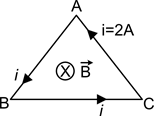A triangular loop in the form of an equilateral triangle is carrying a current of 2A.The loop is placed in a uniform magnetic field of induction 1.5 T, with its plane perpendicular to the magnetic field as shown in figure. Then net force experienced by the loop is

# A triangular loop in the form of an equilateral triangle is carrying a current of 2A.The loop is placed in a uniform magnetic field of induction 1.5 T, with its plane perpendicular to the magnetic field as shown in figure. Then net force experienced by the loop is1. A

2. B

3. C

4. D

Zero

Register to Get Free Mock Test and Study Material

+91

Live ClassesRecorded ClassesTest SeriesSelf Learning

Verify OTP Code (required)

### Solution:

$\stackrel{\to }{\mathrm{F}}=\mathrm{i}\stackrel{\to }{{l}_{e}}×\stackrel{\to }{\mathrm{B}}$.

For any closed current carrying loop, $\stackrel{\to }{{l}_{e}}$= 0.

$\therefore$ F = 0 .